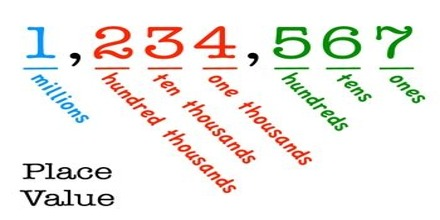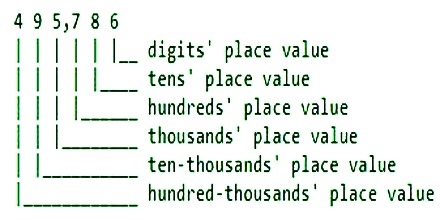Place Values of Addition – Expanded Form

In our decimal number system, the value of a digit depends on its place, or position, in the number. Each place has a value of 10 times the place to its right.The place value is the value of the location of a digit in a number. The place values are determined by how many places the digit lies to the right or the left of the decimal point.

The place values to the left of the decimal point are increasing powers of 10:

• The first place to the left of the decimal point is the ones place, or 100.
• The second place to the left of the decimal is the tens place, or 101.
• The third place to the left of the decimal is the hundreds place, or 102.

Each digit of a number, such as 495,786, has a different place value name.There are 4 sets of one hundred thousand, 9 sets of ten thousand, 5 sets of one thousand, 7 sets of one hundred, 8 sets of ten, and 6 ones in the number 495,786.

Expanded form shows the number expanded into an addition statement. The expanded form of 495,786 is 400,000 + 90,000 + 5,000 + 700 + 80 + 6.